Home | Introduction | Software | Examples | History | People | Bibliography | Applications | Theorems

## Pseudospectra of Toeplitz Matrices and Operators: Banded Matrices

Banded Toeplitz matrices arise in many applications, such as the finite difference discretizations of differential equations. One may wish to know the eigenvalues of such a matrix, for example, to determine whether the discretization of an initial-boundary value problem is stable.

Suppose the Toeplitz matrix T is defined entrywise,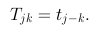The coefficients tj define a function a by the Laurent series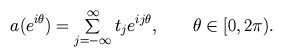If the Toeplitz matrix T is banded, then there exists some j0 such that tj=0 whenever |j|>j0. This implies that the function a is a trigonometric polynomial.

The text of Böttcher and Silbermann [BS99] provides a comprehensive introduction to the subject. Widom's survey article [Wid65] is another good starting point. We use the notation TN to denote the N-by-N finite section of the infinite dimensional Toeplitz matrix T.

The eigenvalues of finite banded Toeplitz matrices lie on curves in the complex plane that have been characterized by Schmidt and Spitzer [SS60]. In contrast, the spectrum of the corresponding infinite dimensional Toeplitz operator is the set of points in the complex plane that a(T) encloses with non-zero winding number; see Theorem 1.17 of [BS99]. The limit of the spectrum of banded Toeplitz matrices as the dimension goes to infinity is thus typically very different from the spectrum of the limit.

This uncomfortable situation can be resolved by considering the behavior of pseudospectra. Though the eigenvalues of the finite Toeplitz matrices may fall on curves in the complex plane, Landau [Lan75], Reichel and Trefethen [RT92b], and Böttcher [Böt94] have observed that the resolvent norm ||(zI-TN)-1|| grows exponentially in the matrix dimension for all z in the interior of the spectrum of the corresponding infinite dimensional operator. (As a result, it is difficult to accurately compute eigenvalues of non-symmetric banded Toeplitz matrices even for matrices of relatively modest dimensions, and this signals a warning against basing analysis of finite Toeplitz matrices based on eigenvalues alone.) Furthermore the above cited authors also concluded that the pseudospectra of TN converge to the pseudospectra of T.

We now turn to several examples of computed pseudospectra of finite Toeplitz matrices. A common theme to all these calculations is the distance between the pseudospectral boundary and the eigenvalues even for small values of epsilon.

Our first example is the simplest non-symmetric Toeplitz matrix, the shift operator a(t)=t. The infinite dimensional version is a classic example in operator theory, and finite shift operators arise in linear algebra in the Jordan cannonical form. This matrix was the first example in the early survey [Tre92].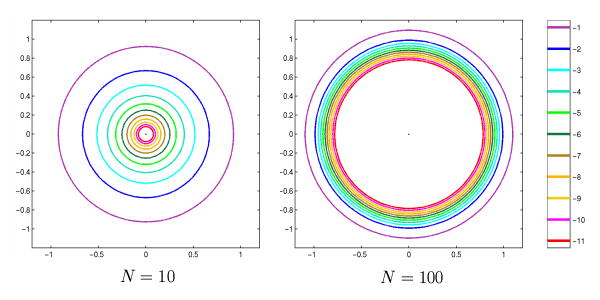Figure 1. Spectrum and epsilon-pseudospectra for the shift matrix of dimension 10 (left) and dimension 100 (right). Note the high degree of non-normality present in the small matrix, and how this non-normality explodes as the dimension is increased.

The "Grcar matrix" is another famous example. Like the last example, it appears in the survey [Tre92] and has become a popular test problem in papers on the computation of pseudospectra. The matrix is determined by the symbol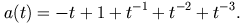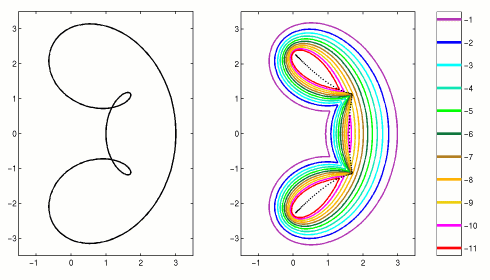Figure 2. Grcar matrix of dimension N=100. The map of the symbol, a(T) (left) and the spectrum and epsilon-pseudospectra (right).

The pseudospectra of our next example resemble petals of a flower, as seen in Figure 3. The corresponding symbol is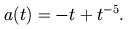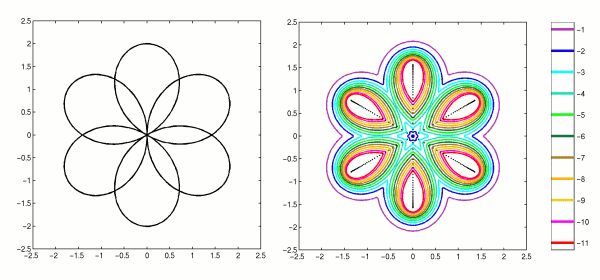Figure 3. "Daisy" matrix of dimension N=200. The map of the symbol, a(T) (left) and the spectrum and epsilon-pseudospectra (right).

Our final example, in Figure 4, is from a banded Toeplitz matrix with a hole in the spectrum of the corresponding infinite dimensional operator. Notice that the pseudospectra do not grow rapidly in the interior region where the winding number of a(T) is zero.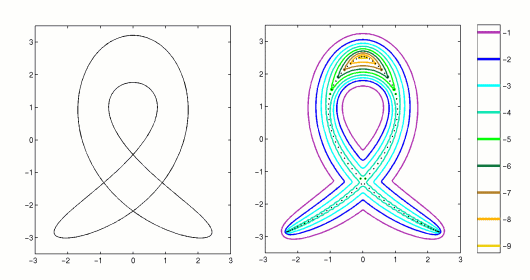Figure 4. Truncation of a Toeplitz operator with a hole in the spectrum. The map of the symbol, a(T) (left) and the spectrum and epsilon-pseudospectra for a matrix of dimension N=100(right).

# Supplemental Bibliography

References to works included in the Pseudospectra Bibliography are presented as links above, and are not repeated here.
 [SS60] P. Schmidt and F. Spitzer The Toeplitz matrices of an arbitrary Laurent polynomial Math. Scand. 8 (1960), 15--38. MR 23 #A1977
 [Wid65] H. Widom Toeplitz matrices In Studies in Real and Complex Analysis, Studies in Mathematics, vol. 3. I. I. Hirschman, Jr., ed. Mathematical Association of America, Buffalo, N.Y., 1965.